LR + 海量人工特征 是业界流传已久的做法，这个方法由于简单、可解释性强，因此在工业界得到广泛应用，但是这种做法依赖于特征工程的有效性，也就是需要对具体的业务场景有深刻的认识才能提取出好的特征。

### 原理

LR 是一个很简单的线性模型，其输出的值可认为是事件发生($y=1$)的概率，即输出值如下式所示

$$h(x) = p(y=1|x) = \sigma(w^Tx+b)$$

$$p(y|x) = h(x)^y(1-h(x))^{1-y}$$

$$\max \prod_{i=1}^np(y_i|x_i)$$

$$\min -\sum_{i=1}^ny_i\log h(x_i)+(1-y_i)\log (1-h(x_i))$$

### 一些问题

1. LR 的输出为什么可以被当做是概率值？

$$\phi = 1/(1+e^{-\eta})$$

2. 为什么 L1 正则项能够带来稀疏性？

1）$w_i<0$ 时, $L+C|w_i|$ 的导数为 $L’- C$
2) $w_i>0$时, $L+C|w_i|$ 的导数为 $L’+C$

3.连续特征为什么需要离散化？

1) 稀疏向量内积乘法运算速度快，计算结果方便存储
2) 离散化后的特征对异常数据有很强的鲁棒性：比如一个特征是年龄>30是1，否则0。如果特征没有离散化，一个异常数据“年龄300岁”会给模型造成很大的干扰；
3) 逻辑回归属于广义线性模型，表达能力受限；单变量离散化为N个后，可以通过 one-hot 编码为每个变量设置单独的权重，相当于为模型引入了非线性，能够提升模型表达能力，加大拟合
4) 离散化后可以进行特征交叉，由M+N个变量变为M*N个变量，进一步引入非线性，提升表达能力；
5) 特征离散化后，模型会更稳定，比如如果对用户年龄离散化，20-30作为一个区间，不会因为一个用户年龄长了一岁就变成一个完全不同的人。当然处于区间相邻处的样本会刚好相反，所以怎么划分区间要取决于具体的场景

4.1 为什么要对 categorical 特征做 One-hot 编码后再输入 LR？

## LS-PLM(Large Scale Piece-wise Linear Model)

LS-PLM(也叫作 MLR, Mixture of Logistics Regression)是阿里妈妈在 2017 年在论文 Learning Piece-wise Linear Models from Large Scale Data for Ad Click Prediction 中公开的，但是模型早在 2012 年就在阿里妈妈内部使用。这个模型在 LR 基础上进行了拓展，目的是为了解决单个 LR 无法进行非线性分割的问题。

### 原理

LR 是一个线性模型，模型会在数据空间中生成一个线性分割平面，但是对于非线性可分的数据，这一个线性分割面显然无法正确分割这些数据。以下图为例（摘自上面的论文），A）为一组非线性训练数据的正负样本分布；对于该问题，LR会生成 B）中的分割平面，C) 图展示的 LS-PLM 模型 则取得了较好的效果。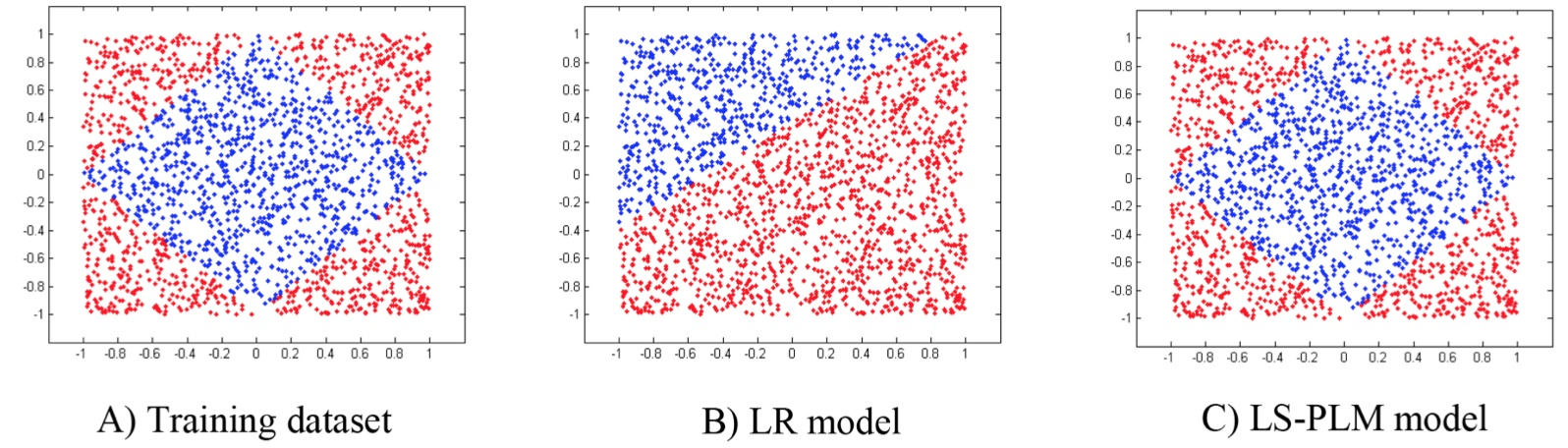LS-PLM 也是采用这个思想的，不够这里不是划分场景，而是划分数据，通过将数据划分不同的region、然后每个region分别建立 LR。

$$p(y=1|x) = g ( \sum_{j=1}^m \sigma(\mu_j^T x)\eta(w_j^Tx))$$

• $m$ : region 的个数(超参数：一般是10~100)
• $\Theta={\mu_1,\dots,\mu_m, w_1,\dots,w_m }$: 表示模型的参数，需要训练
• $g(\cdot)$：为了让模型符合概率定义(概率和为1)的函数
• $\sigma(\cdot)$：将样本分到 region 的函数
• $\eta(\cdot)$：在 region 中划分样本的函数

$$p(y=1|x) = \sum_{i=1}^m \frac{e^{\mu_i^Tx}}{\sum_{j=1}^m e^{\mu_j^Tx}}\frac{1}{1+e^{-w_i^Tx}}$$

$$||\Theta||_{2,1} = \sum_{i=1}^d \sqrt {\sum_{j=1}^m(\mu_{ij}^2+w_{ij}^2)}$$

MLR 适用的场景跟 LR 一样，也是适用于高纬稀疏特征作为输入。

## GBDT+LR(Gradient Boost Decision Tree + Logistic Regression)

GBDT + LR 是 FaceBook 在这篇论文 Practical Lessons from Predicting Clicks on Ads at Facebook 中提出的，其思想是借助 GBDT 帮我们做部分特征工程，然后将 GBDT 的 输出作为 LR 的输入。

### 原理

1. 连续变量切分点如何选取？
2. 离散化为多少份合理？
3. 选择哪些特征交叉？
4. 多少阶交叉，二阶，三阶或更多？

GBDT 最早在这篇论文 Greedy Function Approximation：A Gradient Boosting Machine 中提出；
GBDT 中主要有两个概念：GB(Gradient Boosting)和DT(Decision Tree)，Gradient Boosting 是集成学习中 boosting 的一种形式，Decision Tree 则是机器学习中的一类模型，这里不对这两者展开，只讲述在 GBDT 中用到的内容。关于决策树的介绍可参考这篇文章 决策树模型 ID3/C4.5/CART算法比较

1. 假设切分后左子树有 $m$ 个样本，右子树有 $n$ 个
2. 计算左子树样本的目标值的均值为 $y_m = \frac{1}{m}\sum_{i=1}^{m}y_i$, 同样计算右子树样本的目标值的均值为 $y_n = \frac{1}{n}\sum_{j=1}^{n}y_j$
3. 平方误差和为 $L = \sum_{i=1}^m(y_i - y_m)^2 + \sum_{j=1}^n(y_j - y_n)^2$
4. 对于每一个可能的切分值，我们都可计算其平方误差和 $L$，选择使得 $L$ 最小的切分点即可。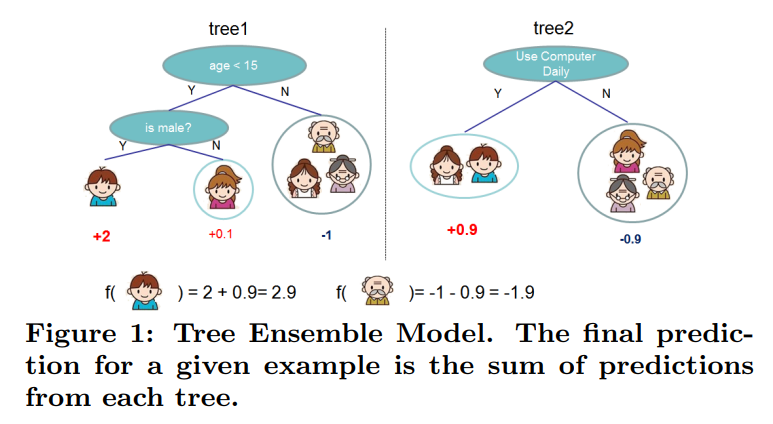$$F(x) = \sum_{t=1}^Tf_t(x)$$

$$y_{ik} = y_i - \sum_{t=1}^{k-1}f_t(x_i)$$GBDT+LR 方案中每棵决策树从根节点到叶节点的路径，会经过不同的特征，此路径就是特征组合，而且包含了二阶，三阶甚至更多，因此输出的 one-hot 特征是原始特征进行交叉后的结果。而且每一维的特征其实还是可以追溯出其含义的，因为从根节点到叶子节点的路径是唯一的，因此落入到某个叶子节点表示这个特征满足了这个路径中所有节点判断条件。

GBDT 适用的问题刚好与 LR 相反，GBDT 不适用于高纬稀疏特征，因为这样很容易导致训练出来的树的数量和深度都比较大从而导致过拟合。因此一般输入GBDT 的特征都是连续特征。

1) 离散特征不直接输入到 GBDT 中进行编码，而是做 one-hot 编码后直接输入到 LR 中即可；对于连续特征，先通过 GBDT 进行离散化和特征组合输出 one-hot 编码的特征，最后结合这两种 one-hot 特征直接输入到 LR。大致框架如下所示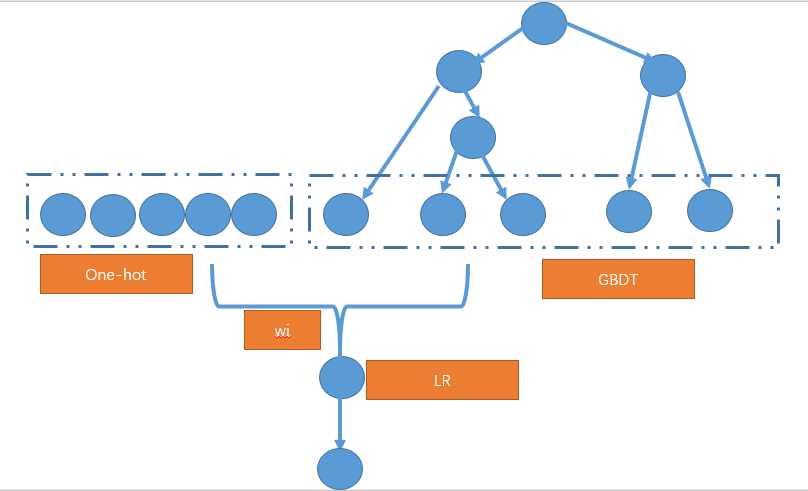2) 将离散的特征也输入 GBDT 进行编码，但是只保留那些出现频率高的离散特征，这样输入 GBDT 中的 one-hot 特征的维度会遍地，同时通过 GBDT 也对原始的 one-hot 特征进行了组合和交叉。

### 一些问题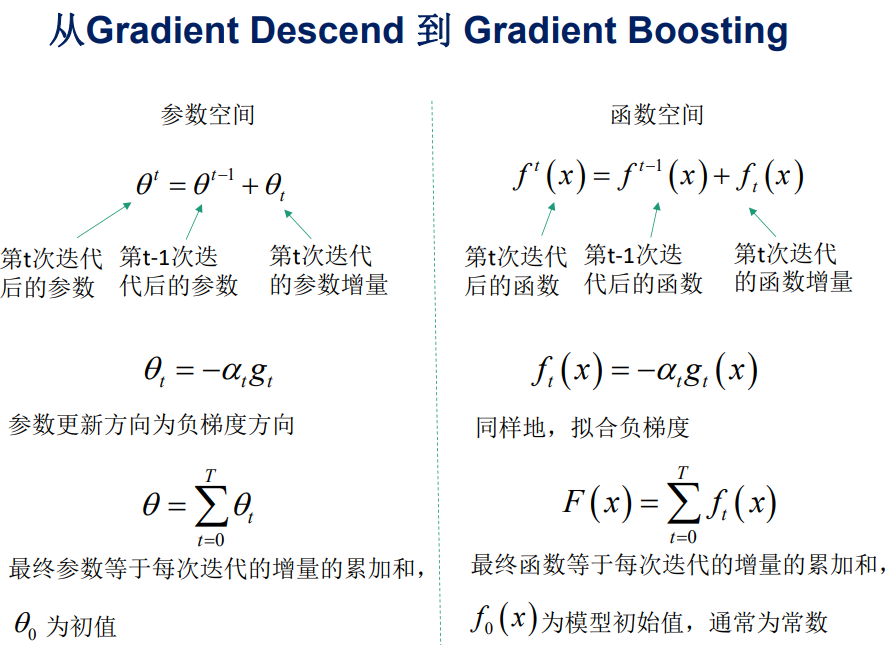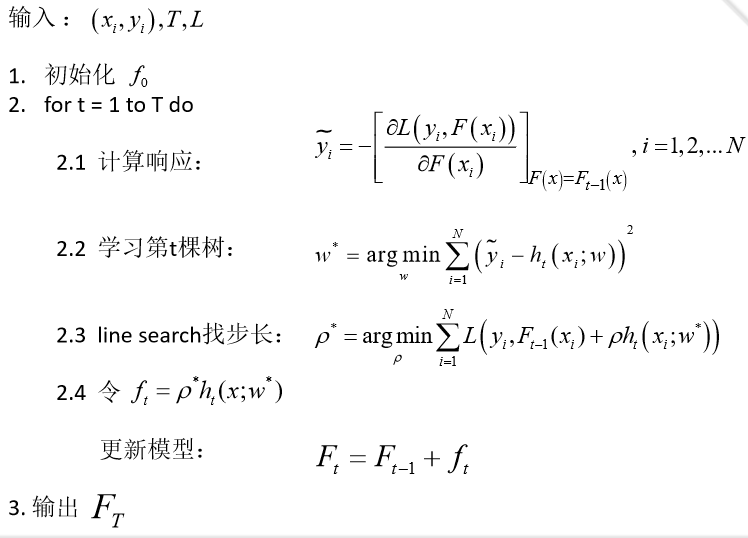$$\tilde{y_i} = y_i - F(x_i)$$

2. GBDT 怎么处理分类问题？

$$p_{c} = \frac{\exp(f_{j}(x))}{ \sum_{i=1}^{k}{exp(f_{k}(x))}}$$

## FM(Factorization Machine)

FM（Factorization Machine）是于2010年在论文 Factorization Machines 中提出，旨在解决稀疏数据下的特征组合问题。其思想是对组合特征的参数所构成的参数矩阵进行矩阵分解，从而得到每个原始特征的隐向量表示，更新特征的隐向量对数据的稀疏性具有鲁棒性。关于 FM 和 FFM ，美团点评这篇文章：深入FFM原理与实践 其实已经写得很详细了，本文主要参考该文章进行修改。

### 原理

FM 可以认为是在 LR 的基础上加入特征的二阶组合，即最多有两个特征相乘，则模型可表示成如下形式

$$y(\mathbf{x}) = w_0+ \sum_{i=1}^n w_i x_i + \sum_{i=1}^n \sum_{j=i+1}^n w_{ij} x_i x_j$$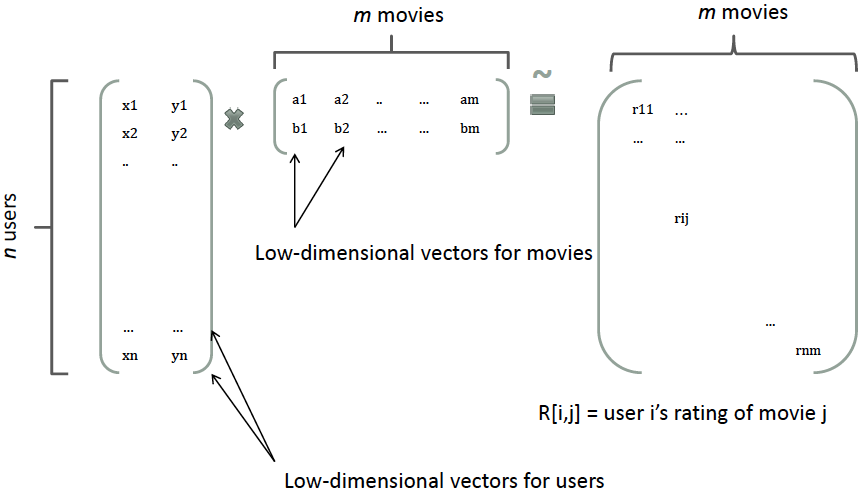$$y(\mathbf{x}) = w_0+ \sum_{i=1}^n w_i x_i + \sum_{i=1}^n \sum_{j=i+1}^n x_i x_j$$

$$\sum_{i=1}^n \sum_{j=i+1}^n \langle \mathbf{v}_i, \mathbf{v}_j \rangle x_i x_j = \frac{1}{2} \sum_{f=1}^k \left(\left( \sum_{i=1}^n v_{i, f} x_i \right)^2 - \sum_{i=1}^n v_{i, f}^2 x_i^2 \right)$$

### 开源实现

FM 在 github 上有单机版本的开源实现 fastFMpyFM， fastFM 是一个学术项目，发表了相关论文 fastFM: A Library for Factorization Machines, 对 FM 进行了拓展；同时我们前面提到的腾讯的PS Angel 中也实现了这个算法，可参考这里

## FFM(Field-aware Factorization Machine)

FFM 发表于论文 Field-aware Factorization Machines for CTR Prediction， 是台大的学生在参加 2012 KDD Cup 时提出的，这个论文借鉴了论文 Ensemble of Collaborative Filtering and Feature Engineered Models for Click Through Rate Prediction 中的 field 的 概念，从而提出了 FM 的升级版模型 FFM。

### 原理

$$y(\mathbf{x}) = w_0 + \sum_{i=1}^n w_i x_i + \sum_{i=1}^n \sum_{j=i+1}^n \langle \mathbf{v}_{i, f_j}, \mathbf{v}_{j, f_i} \rangle x_i x_j$$

FFM 适用的场景跟 FM 和 LR 一样，适用于输入的特征是高维稀疏特征

### 开源实现

FFM 最早的开源实现是台大提供的 libffm，去年开源的 xlearn 中也提供了该算法的实现，提供的 api 比 libffm 更加友好。